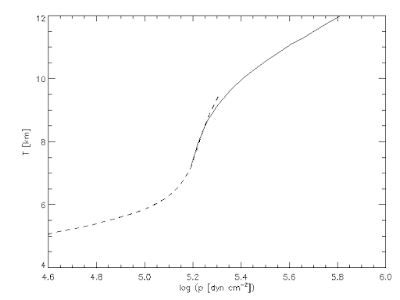## Sunday, 12 July 2015

### Notes on some basic quantities, units and constants: Part II

Mass fractions

The mass fraction of H, He and the metals is a quick way to specify the chemical composition of the stellar plasma. The mass fraction of hydrogen is defined as ratio of the mass of hydrogen particles and the total mass of all particles (in a given volume): $$X = \frac{M_\mathrm{H}}{M}= \frac{\rho_\mathrm{H}}{\rho},$$ where $\rho_\mathrm{H}$ and $\rho$ are the respective (mass) densities. The hydrogen mass density is equal to the hydrogen number density ($n_\mathrm{H}$) times the mass of one hydrogen particle $m_{\mathrm{H}} = A_{\mathrm{H}}\,m_{\mathscr{A}}$, thus: $$X = \frac{M_\mathrm{H}}{M}= \frac{n_\mathrm{H}\,m_{\mathrm{H}}}{\rho}= \frac{n_\mathrm{H}\,A_{\mathrm{H}}\,m_{\mathscr{A}}}{\rho}.$$ Similar to that, for helium and the metals we define: $$Y = \frac{M_\mathrm{He}}{M}= \frac{n_\mathrm{He}\,m_{\mathrm{He}}}{\rho}= \frac{n_\mathrm{He}\,A_{\mathrm{He}}\,m_{\mathscr{A}}}{\rho},$$ $$Z = \frac{M_\mathrm{metals}}{M}= \frac{n_\mathrm{metals}\,m_{\mathrm{metals}}}{\rho} = \frac{\sum_{i=3} n_\mathrm{i}\,A_{\mathrm{i}}\,m_{\mathscr{A}}}{\rho},.$$

## Saturday, 11 July 2015

### Notes on some basic quantities, units and constants: Part I

There is a definition of mole as a unit that every student learns at very elementary level. Although mole is one of the seven base units of the International System, it is a bit specific and sometimes creates confusion. Here is my attempt to clarify the concept of mole and to derive in one place some useful relations used in the radiative transfer and atmospheric modeling. The first version of these notes I wrote for a group of students at the University of Belgrade many years ago. I still use them as a personal reminder.

Counting "Elementary particles"

For the solar/stellar plasma, the "elementary" particles are atoms, ions (positive or negative), free electrons and molecules. In the very cool atmospheres there are dust particles as well. In the solar atmosphere dust can be completely neglected. Regarding the chemical composition, the atmospheric plasma is made out of hydrogen, helium and the metals (all other elements). There is no nuclear reactions and thus the total number density of nuclei per atomic specie is constant with time.

It is important to distinguish between the number of free atoms and the total number of atoms including those bound in the molecules. The former I denote as $N_{\mathrm{a}}^{\mathrm{free}}$, the latter as $N_{\mathrm{a}}^{\mathrm{tot}}$. The total number of atoms is identical to the number of atomic nuclei. The total number of molecules is $N_{\mathrm{m}}$.

The total number of particles $N$ is therefore:
$$N = N_\mathrm{e} + N_{\mathrm{a}}^{\mathrm{free}} + N_{\mathrm{m}},$$ or when there is no molecules $$N = N_\mathrm{e} + N_{\mathrm{a}}^{\mathrm{free}} = N_\mathrm{e} + N_\mathrm{H} + N_\mathrm{He} + N_\mathrm{\mathrm{metals}},$$ where $\mathrm{e}$, $\mathrm{m}$, $\mathrm{H}$ and $\mathrm{He}$ stand for the electrons, the molecules, hydrogen, helium and $\mathrm{metals}$ refer to all other elements together. The contribution of the metals can be further divided into the contributions of the individual elements.

## Friday, 15 May 2015

### 1D Solar Models in IDL: Spruit's Convection Model

The semi-empirical models of the solar atmosphere rely on the observed intensities and, therefore, they cannot say much about the invisible convection zone below the surface. Spruit (1974SoPh...34..277S) constructed a 1D model of the solar convection zone using the mixing-length theory with 4 free parameters. The model is constructed so that it matches the HSRA model (Gingerich et al, 1971SoPh...18..347G) of the solar atmosphere. The model of Spruit is sometimes used to initiate the convection simulations with 3D numerical codes.Fig 1. The HSRA model atmosphere (dashed) and Spruit's model of convective zone (solid). This is reproduced after Fig.3 of Spruit's paper.

## Sunday, 12 April 2015

### Atlantis over Tenerife

Japanese astronaut Sochi Noguchi took this iconic picture of the Space Shuttle Atlantis over the Atlantic Ocean and the island of Tenerife. The picure was taken from the International Space Station on Sunday, May 16 2010 at 10:28 am EDT (1428 GMT) while the Atlantis was getting ready for docking. At that time the ISS was about 350 km above the ground. This was the flight before the last one for the shuttle. In the highest resolution, the white towers of the solar telescopes at the Observatorio del Teide (IAC) are visible between the clouds. To help your eyes, I took a snapshot from the Google Maps: look for the distinctive dark patches in the NASA picture, the towers are tiny white dots just above them.Credit: NASA/Sochi Noguchi (click for hi-res)Credit: Google Maps (click to go to the interactive map)

## Saturday, 28 February 2015

### 1D Solar Atmosphere Models in IDL: Gingerich et al (HSRA)

The Harvard-Smithsonian Reference Atmosphere (HSRA) by Gingerich et al (1971, 1971SoPh...18..347G) is another widely used semi-empirical model of the idealized plane-parallel solar atmosphere in hydrostatic equilibrium. This model also includes the chromosphere where the hydrogen ionization is solved using the statistical equilibrium equations. The model is still often used as the reference solar atmosphere in 1D and its number of citations steadily grows (> 820 so far). The paper is very clearly written and it's a very recommendable reading especially if you use this model. Beside the model itself, there is several tables with computed intensities, brightness temperature, optical depths at different wavelengths, etc.